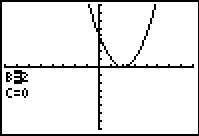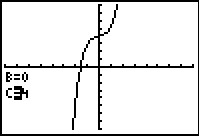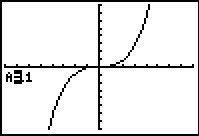### Mathematics lessons for IB® Diploma Programme

Applications and Interpretation | Functions## Just Move It

### Activity Overview

Students explore translations, compressions and stretches of the parent function graph f(x) = x² and f(x) = x³.

## Key Steps## Step 1

In this activity, students explore transformations of graphs. Modifications are made to the input and output of parent functions. In the first problem, they will begin with the transformation of f(x) → f(x–h), by changing the input values.## Step 2

Students will then complete the transformation of ff(x)→f(x)+k, by changing the output values. They will confirm their observations by exploring the transformation of h and k together.## Step 3

If time permits, students can explore the transformation of ff(x)→af(x). They will use fractions as well as whole numbers in their investigation. Students will also compare negative a-values to positive a-values.